# Multiplying 4-Digit by 1-Digit Numbers

Times up!
Times up! You may finish answering the current question and then proceed to your score!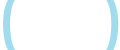Sorry, you have used all of your available hints for this lesson.
Practice Limit Reached

You've reached your daily practice limit of 12 questions.

Award InformationAwarded toQuestion HintChoose Family Member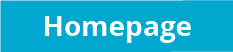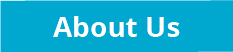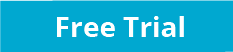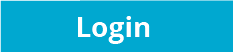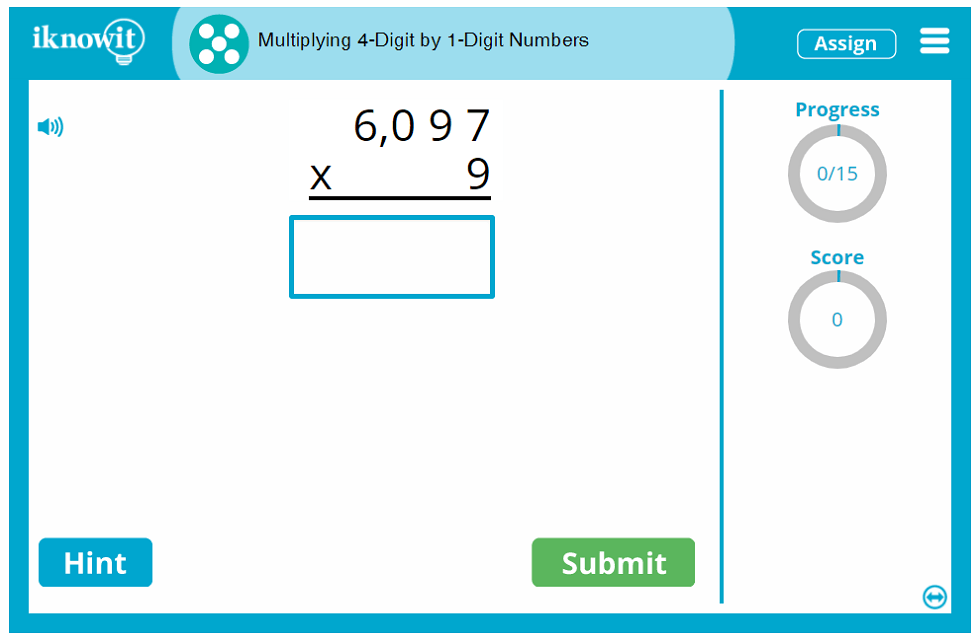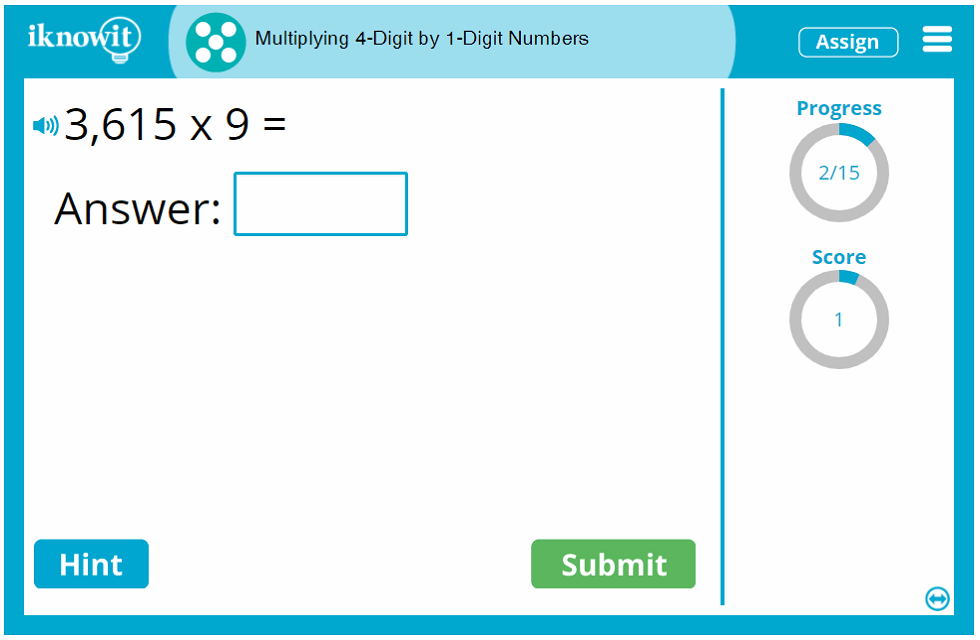## Interactive Math Activity - Multiplying 4-Digit by 1-Digit Numbers

Challenge your students to sharpen their multiplication skills with this interactive math activity geared toward fifth grade. Children will practice multiplying four-digit by one-digit numbers in this online multiplication lesson. Math problems are presented as vertical multiplication problems, horizontal multiplication problems, and multiplication word problems. When your students complete this online math game, they will gain confidence and proficiency in their advanced multiplication skills.

Your students will solve a variety of multiplication problems in this interactive math lesson. Here are a couple examples of the word problems students may be required to solve: "There are four walruses at the aquarium, and each one weighs 2,043 pounds. What is the combined weight of the walruses at the aquarium?" or "Each one of the Snow Goose Inn hotels has 1,776 rooms. There are 8 hotel locations. What is the total number of rooms at all Snow Goose Inn hotels?" Or students may be asked to solve multiplication problems like these: "5,499 x 2 =" and "7,497 x 5=" Irregardless of the type of multiplication problem presented, students can use the "Hint" option if they need a little extra help solving a problem. The hint will offer the first step in solving the multiplication problem, "Multiply the ones," along with a friendly reminder: "Remember to regroup when necessary." When students answer a question incorrectly, a detailed explanation page will show the right answer with easy-to-follow step-by-step instructions for obtaining the solution. Students will move through the lesson having learned from past mistakes.

Several built-in lesson features available in this fifth-grade multiplication lesson, as well as all math practice activities on "I Know It," help students make the most of their math practice session. For example, students can view how many questions they have answered so far in the lesson using the "Progress Tracker" tool. They can also see how many points they have earned for correct answers using the "Score Tracker" feature. If students would like to hear the question read out loud to them in a clear voice, they can click the speaker icon in the upper-left corner of their practice screen. This is an excellent resource for ESL/ELL students and for students who excel at auditory learning! As you can see, every "I Know It" math lesson is designed with your students' success in mind.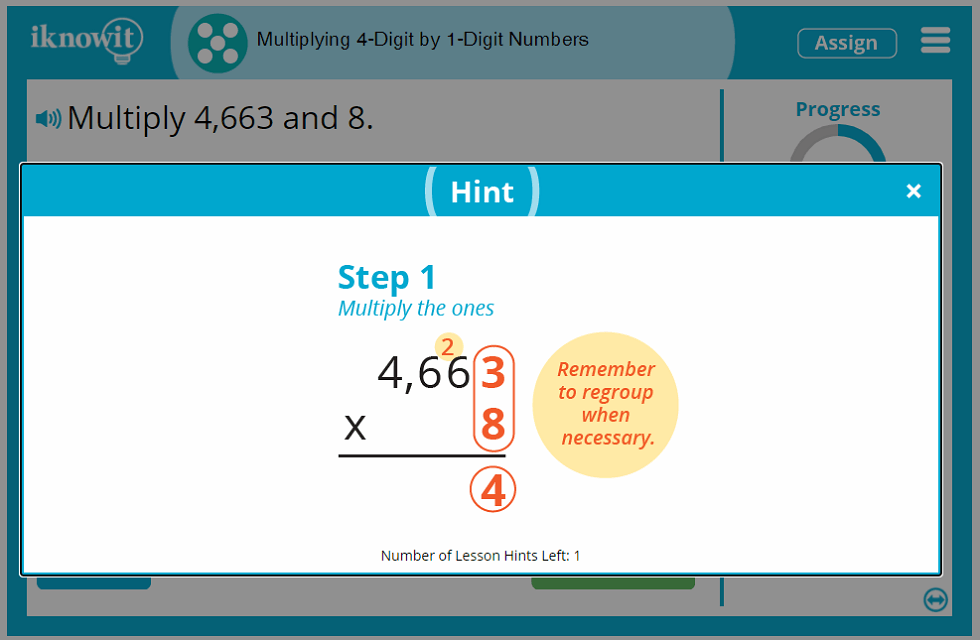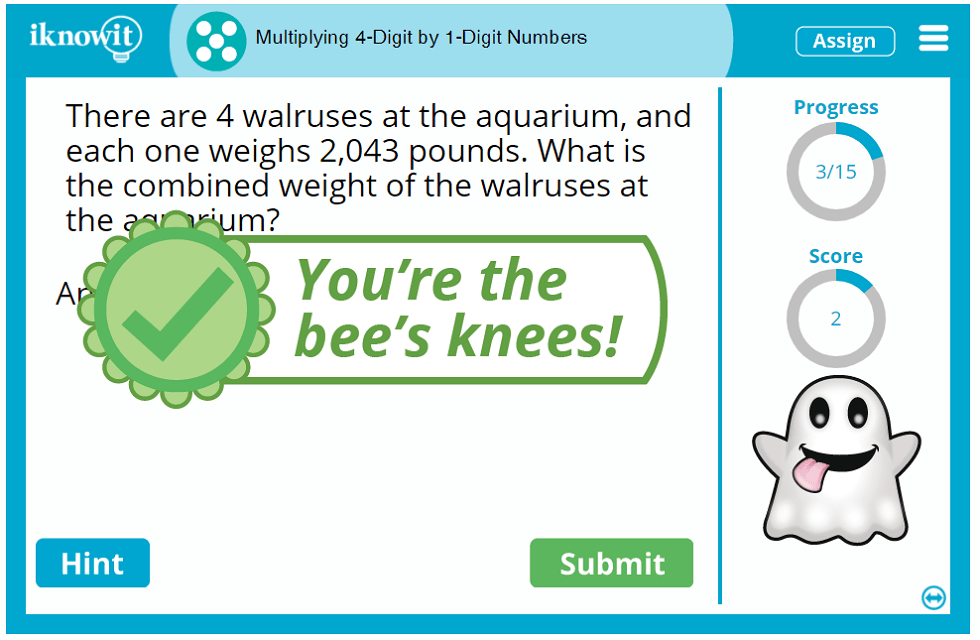## 'I Know It' - The Right Choice for Teachers and Students

Elementary teachers and homeschool educators just love using the "I Know It" online math program with their students! Our math lesson library covers dozens of popular elementary math topics from kindergarten through fifth grade—from counting coins to multiplying 4-digit by 1-digit numbers! Teachers appreciate the variety and quality of math topics we cover. Each math lesson on our website is thoughtfully written by elementary teachers to meet at least one Common Core Standard. Math lessons are organized on the website by grade level and topic, making it easy for you to find and assign lessons in just a few clicks.

Students, too, love sharpening their math skills using the "I Know It" math program! Kids think our online math games are tons of fun! From the cute animated characters cheering on students in their math practice to the math awards kids can earn for mastering new math skills, math practice isn't a chore with "I Know It" - it's an exciting challenge!

We hope you and your students will try out this fifth-grade multiplication activity and discover all there is to love about "I Know It"! Be sure to check out our hundreds of other fifth grade math topics too!

## Test the Waters or Dive Right In

Looking to test the waters to see if the "I Know It" math program is right for you and your class? Sign up for our free thirty-day trial offer (no credit card required) and explore any of the math practice activities on our website for a full thirty days! We're confident you and your students will love the difference interactive math practice can make—when your free trial runs out, we hope you will consider joining the "I Know It" community as a member so you can continue to enjoy the benefits of our program for a full calendar year. We have the perfect membership option for everybody! Whether you're looking for a family membership, individual teacher membership, or school or school district package, we have an option tailor-made for you! Check out our membership page to learn more today: https://www.iknowit.com/order.html.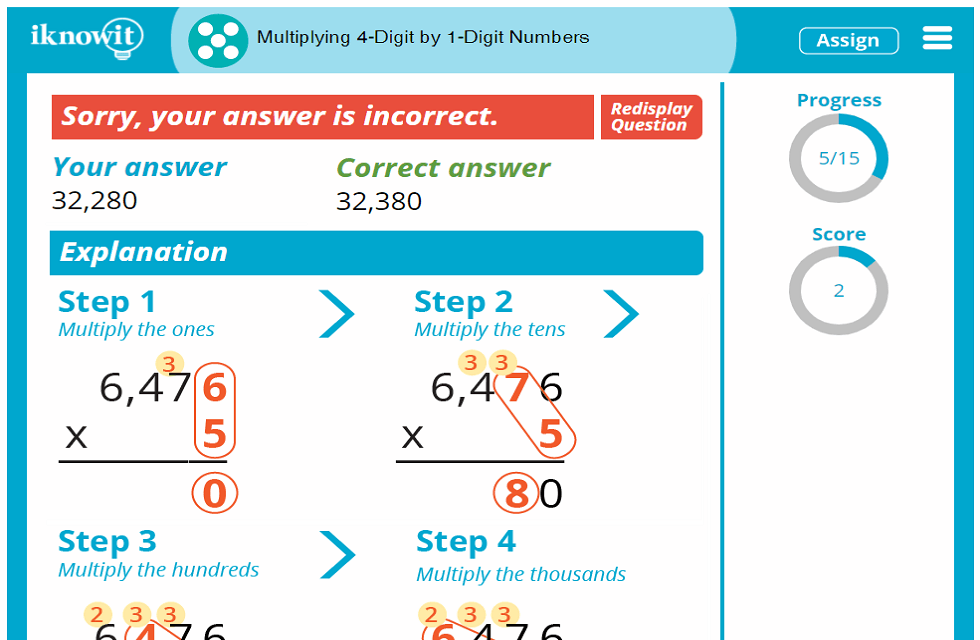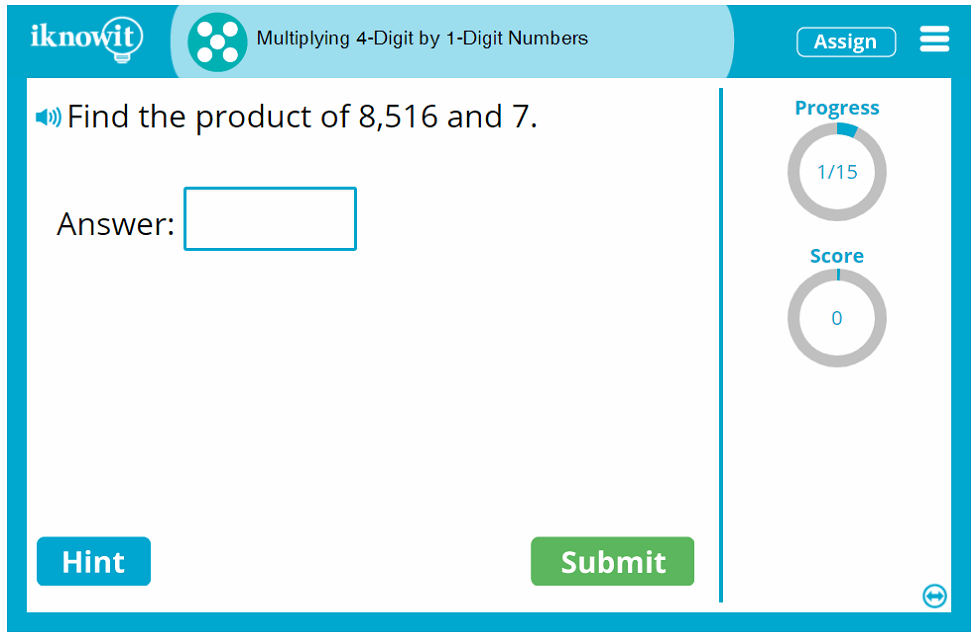## Level

This interactive math lesson is classified as Level E. It may be ideal for a fifth-grade class.

## You might also be interested in...

Multiplying 2 and 3-Digit Numbers by 1-Digit Numbers (Level E)
In this fifth grade-level math lesson, students will practice multiplying 2 and 3-digit numbers by 1-digit numbers. Questions are presented as word problems, vertical multiplication problems, and horizontal multiplication problems.

Multiplying 2-Digit by 2-Digit Numbers (Level E)
In this math lesson geared toward fifth grade, students will practice multiplying 2-digit by 2-digit numbers. Questions are presented as word problems, vertical math problems, and horizontal math problems.